Class 10 Science: CBSE Sample Question Paper (2019-20) - 10

# Class 10 Science: CBSE Sample Question Paper (2019-20) - 10 Notes | Study CBSE Sample Papers For Class 10 - Class 10

## Document Description: Class 10 Science: CBSE Sample Question Paper (2019-20) - 10 for Class 10 2022 is part of CBSE Sample Papers For Class 10 preparation. The notes and questions for Class 10 Science: CBSE Sample Question Paper (2019-20) - 10 have been prepared according to the Class 10 exam syllabus. Information about Class 10 Science: CBSE Sample Question Paper (2019-20) - 10 covers topics like and Class 10 Science: CBSE Sample Question Paper (2019-20) - 10 Example, for Class 10 2022 Exam. Find important definitions, questions, notes, meanings, examples, exercises and tests below for Class 10 Science: CBSE Sample Question Paper (2019-20) - 10.

Introduction of Class 10 Science: CBSE Sample Question Paper (2019-20) - 10 in English is available as part of our CBSE Sample Papers For Class 10 for Class 10 & Class 10 Science: CBSE Sample Question Paper (2019-20) - 10 in Hindi for CBSE Sample Papers For Class 10 course. Download more important topics related with notes, lectures and mock test series for Class 10 Exam by signing up for free. Class 10: Class 10 Science: CBSE Sample Question Paper (2019-20) - 10 Notes | Study CBSE Sample Papers For Class 10 - Class 10
 1 Crore+ students have signed up on EduRev. Have you?

Class-X
Science-086
SAMPLE QUESTION PAPER 2019-20

TIME: 3 Hrs.
M.M: 80

General Instructions:
1. The question paper comprises three sections - A, B and C. Attempt all the sections.
2. All questions are compulsory.
3. Internal choice is given in each section.
4. All questions in Section A are one - mark questions comprising MCQ, VSA type and
assertion-reason type questions. They are to be answered in one word or in one sentence.
5. All questions in Section B are three - mark, short - answer type questions. These are to be answered in about 50 - 60 words each.
6. All questions in Section C are five - mark, long - answer type questions. These are to be answered in about 80 - 90 words each.
7. This question paper consists of a total of 30 questions.

Section A

Q 1. Newly formed DNA copies may not be identical at times. Give one reason.
OR
Name the hormone in human which regulates carbohydrate, protein and fat metabolism in the body. Mention the site where it is synthesized.    (1 Mark)
Ans
:  If there is an error in DNA copying or imitation, then newly formed DNA copies may not be identical at times.
OR
The hormone is thyroxin.
The site where it Is synthesized is thyroid gland.

Q 2. What is hydrogenation? What is its industrial application ?    (1 Mark)
Ans: The addition of hydrogen to unsaturated hydrocarbons in the presence of catalyst like palladium, platinum, nickel, etc., to give saturated hydrocarbons is called hydrogenation.
example: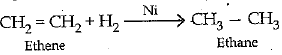This reaction is used for hydrogenation of liquid vegetable oil to get vanaspati ghee.

Q.3. Which of the following experimental set up is correct for verification of Ohm’s law?    (1 Mark)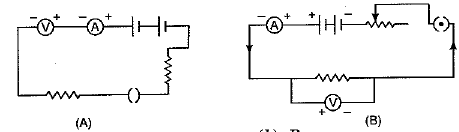(a) A
(b) B
(c) Both A and B
(d) Neither A nor B

Ans: (b) Experimental set up B is correct for verification of Ohm’s law

Q 4. For Q. Nos. 4(i)-4(iv) are based on the table given below. Study these table related to answer the questions that follow :    (1 Mark)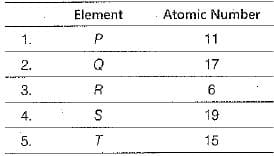Answer the questions given below :
4. (i) Which of the given elements can be cut with a knife?    (1 Mark)
4. (ii) Which element can form an ionic compound with Q?    (1 Mark)
4.(iii) The allotrope of which of these elements is a good conductor of electricity?    (1 Mark)
(a) P
(b) Q
(c) R

(d) S
4. (iv) Which pair of elements are stored in kerosene?    (1 Mark)
(a) P and S
(b) Q and R
(c) R and T
(d) Q and T

Ans: 4. (i) Element P (Na) can be cut with a knife.
4. (ii) Element P (Na) can form an ionic compound with 0 (Cl).
4. (iii) (c) The allotrope of element R (C), that is graphite, is a good conductor of electricity.
4. (iv) (a) The elements P and \$ (Na and K) are stored in kerosene.

Q 5. In the double displacement reaction between aqueous potassium iodide and aqueous lead nitrate, a yellow precipitate of lead iodide is formed. While performing the activity, if lead nitrate is not available, which of the following can be used in place of lead nitrate ?    (1 Mark)
(a) Lead sulphate (insoluble)
(c) Ammonium nitrate
(d) Potassium sulphate

Ans: (b)
Explanation: Lead acetate can be used in place of lead nitrate because like lead nitrate, it is also a soluble salt in water. The reaction is as follows:
Pb(CH3COO)2 + 2KI→ 2PbI2 + 2CH3COO-K+
Lead sulphate is insoluble in water and will not dissociate into Pb2+ ions, so it cannot be used.

Q 6.  Which of the following terms does not represent electrical power in a circuit?    (1 Mark)
(a) I2 R
(b) IR2
(c) VI
(d) V2/R

Ans: (b)

Q 7. When water is added to concentrated sulphuric acid    (1 Mark)
(a) heat is evolved
(b) heat is absorbed
(c) no change takes place
(d) depends upon the acid
Ans:
(a) heat is evolved

Q 8. The non-metals are either solids or gases, except one which is a liquid. Which of the following non-metal is liquid?    (1 Mark)
(a) Mercury
(b) Carbon
(c) Iodine
(d) Bromine
Ans:
(d) Bromine is the only non-metal which is liquid.

Q 9.  A teacher sets up the stand carrying a convex lens of focal length 15 cm at 42.7 cm mark on the optical bench. He asks four students A, B, C and D to suggest the position of screen on the optical bench so that a distinct image of a distant tree is obtained almost immediately on it. The positions suggested by the students were as :    (1 Mark)
(i) 12.7 cm
(ii) 29.7 cm
(iii) 57.7 cm
(iv) 72.7 cm
The correct position of the screen was suggested by
(a) (i)
(b) (ii)
(c) (iii)
(d) (iv)

Ans: (c)
Explanation: The incident rays coming from the distant tree placed will be parallel to the principal axis. Since the rays parallel to the principal axis, after refraction by convex lens, will pass through the principal focus. Hence, a distinct image will be obtained immediately when distance between screen and lens is equal to focal length.
42.7 cm (position of lens on optical bench) + 15 cm (focal length of lens)= 57.7 (the position of screen on optical bench)

Q.10 .Dramatic changes of body features associated with puberty are mainly because of secretion of:    (1 Mark)
(a) Oestrogen from testes and testosterone from ovary.
(b) Estrogen from adrenal gland and testosterone from pituitary gland.
(c) Testosterone from testes and estrogen from ovary.
(d) Testosterone from thyroid gland and estrogen from pituitary gland.
Ans: (c)

Q 11. Complete the following statements with appropriate answer(s) in the blank spacers) :    (1 Mark)
The junction of optic nerve and the retina in the eye is called__________and __________is possible here.
Ans: blind spot, no vision

Q 12. The positions of four elements X , Y , Z and R in the modern periodic table are shown below which element has the smallest atomic radius?    (1 Mark)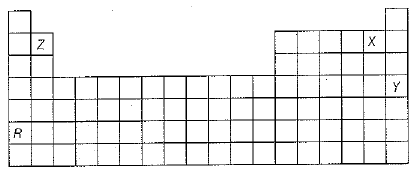(a) X
(b)Y
(c) Z
(d)R

Ans: (a)
Atomic radius decreases along a period and increases down a group in the modern periodic table. Therefore, X has the smallest atomic radii.

Q.13. For question numbers 13 and 14, two statements are given- one labelled Assertion (A) and the other labelled Reason (R). Select the correct answer to these questions from the codes (a), (b), (c) and (d) as given below :
(a) Both A and R are true and R is correct explanation of the assertion.
(b) Both A and R are true but R is not the correct explanation of the assertion.
(c) A is true but R is false.
(d) A is false but R is true.
Assertion (A) : Unisexual flowers have separate male and female flowers.
Reason (R) : Cucumber, pumpkin and watermelon are example of unisexual flowers.
OR
Assertion (A) : Units which make up the nervous system are called neurons.
Reason (R) : Nerve impulses are carried by dendrites towards the cell body    (1 Mark)
Ans: (b) Unisexual flowers have separate j male and female flowers. The example includes cucumber, pumpkin and watermelon.
OR
(b) Both the statements are true. I Nervous system is the system of conducting tissues that receives the stimulus and transmits it to other parts of the body forming a network of nerves. It is involved in receiving information (sensation) and generating responses to that information (motor response). The units which make up the nervous system are called nerve cells or neurons. Nerve impulses are always transmitted across a synapse from the axon terminals of one neuron to the dendrite /cell body of the next neuron.

Q.14. For question numbers 13 and 14, two statements are given- one labelled Assertion (A) and the other labelled Reason (R). Select the correct answer to these questions from the codes (i), (ii), (iii) and (iv) as given below
(i) Both A and R are true and R is correct explanation of the assertion.
(ii) Both A and R are true but R is not the correct explanation of the assertion.
(iii) A is true but R is false.
(iv).  A is false but R is' true.
Assertion : Laws of refraction are valid for all angles on incidence.
Reason: When a.ray of light falls perpendicular on the interface separating two transparent surfaces, it does not undergo refraction.    (1 Mark)
(i) A
(ii) B
(iii) C
(iv) D
Ans:
(iv)

Q.15. Read the Assertion and Reason carefully and then mark the correct option out of the options given below :
(a) If both assertion and reason are true and the reason is the correct explanation of the assertion.
(b) If both assertion and reason are true but reason is not the correct explanation of the assertion.
(c) If assertion is true but reason is false.
(d) If the assertion and reason both are false.
Assertion : All the organic compounds that are obtained from plant and animal sources are being synthesised these days.
Reason : In saturated hydrocarbons, four valencies of carbon are satisfied with the help of single bonds.    (1 Mark)

Ans: (b)

Section B

Q.16. Identify the acid and base which form sodium hydrogen carbonate. Write chemical equation in support of your answer. State whether this compound is acidic, basic or neutral. Also, write its pH value.
OR
What are amphoteric oxides ? Give an example.
Write balanced chemical equations to justify your answer.    (3 Mark)
Ans:
Acid - H2O3
Base - NaOH
NaOH + H2CO3 → NaHCO3 + H2O
Compound is basic in nature.
pH value - ranges between 7 to 10.
OR
Metal oxides showing both acidic and basic nature.
Example: Al2O3/ZnO (or any other)
Al2O3 + 6HCl → 2AlCl3 + 3H2O
Al2O3 + 2NaOH → 2NaAlO2 + H2O

Q.17. Three elements 'X', 'Y' and ‘Z’ having atomic numbers 11, 7 and 6 respectively react with oxygen to form their oxides.
(a) Arrange these oxides in increasing order of their basic nature.
(b) Give reason for your answer.    (3 Mark)
Ans: 11X = 2,8,1
7Y = 2,5
6Z = 2, 5
(a) Y< Z< X
(b) X is metallic in nature hence, its oxide is basic in nature. While Y and Z are non-metals and their oxides are acidic in nature.
(In a period, acidic character and basic character decreases.)

Q.18.  List four functions of the human heart. Why is double circulation necessary in the human body ?    (3 Mark)
Ans: Four functions of human heart are :
(i) It receives deoxygenated blood from the body for oxygenation.
(ii) Sends the blood to the lungs for oxygenation.
(iii) Receives oxygenated blood from the lungs to be supplied to body.
(iv) Sends oxygenated blood to various body parts, so that each cell gets energy.
Double circulation is necessary for human body. It ensures quick and efficient supply of oxygenated blood to all body parts for meeting higher energy needs and for thermoregulation of body in mammals and birds.

Q.19. 'Reproduction is not necessary to maintain the life of an individual, unlike other life processes such as nutrition, respiration or excretion. Still, an individual wastes energy for reproduction. Derive your own conclusion from the above given statement and describe it accordingly.    (3 Mark)
Ans:
Reproduction is the ability of a living organism to produce new individuals similar to them. Like other essential life processes (nutrition, respiration, growth and excretion), reproduction is not essential to maintain the life of an individual. However, it is essential for the purpose of
• continuation of life on the Earth.
• addition of new species.
• replacement of dead organisms.
• transfer of variations from one generation to another.

Q.20. What happens to a beam of white light when it gets refracted through a glass prism ? Which colour deviates the most and the least after refraction through a prism ? What is likely to happen if a second identical prism is placed in an inverted position with respect to the first prism ? Justify your answer.
OR
A student needs spectacles of power - 0.5 D for the correction of his vision.
(i) Name the defect in vision the student is suffering from.
(ii) Find the nature and focal length of the corrective lens.
(iii) List two causes of this defect.    (3 Mark)
Ans: The white light splits into seven colours when it gets refracted through the glass prism (VIBGYOR).
The colour deviates most - Violet
The colour deviates least - Red
Colours disappear and again white light is obtained.

Q.21. Name the hormone synthesised at the shoot tips. How does it help the plant to respond to light ?    (3 Mark)
Ans:
Auxin is synthesised at the shoot tips when growing plant detects light and helps the cells to grow longer.
When light is coming from one side of the plant, auxin diffuses towards the shady side of the shoot. This concentration of auxin stimulates the cells to grow longer on the side of the shoot which is away from light. Thus, the plant appears to bend towards light.

Q.22. List in tabular form three distinguishing features between cerebrum and cerebellum.    (3 Mark)
Ans: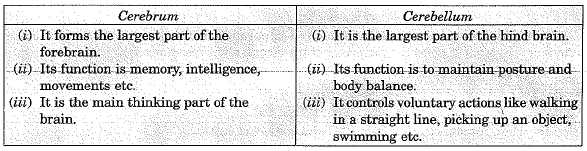Q.23. A household uses the following electric appliances.
(i) A heater of rating 1000 W for 5 hours in each day.
(ii) 5 electric bulbs of rating 100 W each for 5 hours in each day (given, cost of electrical energy Rs. 2.5 per unit).
Find the electricity bill for the household for the month of April.    (3 Mark)

Ans: Total number of units consumed by heater for 5 hours each day in one month,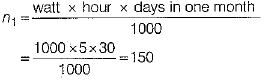Cost of electrical energy consumed by heater in month of April = 150 x 2.5 = Rs. 375
Total number of units consumed by 5 electric bulbs in one month,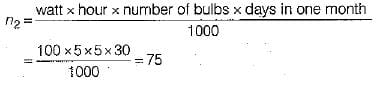Cost of electrical energy consumed by 5 bulbs in one month = 75 x 2.5 = Rs. 187.5
Electricity bill for the month of April = Rs. 375 +Rs. 187.5 = Rs. 562.5
Alternate solution
Total power consumed by electric appliances (heater and 5 bulbs) per day,
P = 1000 + 100 x 5
= 1500 watt
Total number of hours of operation of electric appliances in a month of April,
= 5 x 30 = 150 hours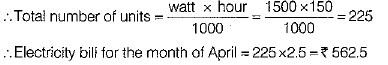Q.24. What are solar cells ? Explain the structure of solar panel. List two principal advantages associated with solar cells.    (3 Mark)
Ans: Solar cells are the devices that convert solar energy of Sun into electricity.
Solar panel consists of a large number of solar cells connected by silver wires. They are made up of special grade silicon. These are mounted on specially designed inclined roof tops so that more solar energy is incident over it.
Advantages of solar cells :
(a) They have no moving parts, require little maintenance and work quite satisfactorily without the use of any focussing device.
(b) They can be set up in remote and inaccessible hamlets or very sparsely inhabited areas.

Section C

Q. 25. (a) Explain why carbon forms covalent bond ? Give two reasons for carbon forming a large number of compounds.
(b) Explain the formation of ammonia molecule.    (5 Mark)

Ans: (a) Carbon has electronic configuration 2, 4. It could gain four electrons forming C+4 anion or lose 4 electrons to form C+4 cation. Both are not possible due to energy considerations.
Carbon overcomes this problem by sharing electrons and forming covalent compounds.
Two reasons for forming large number of compounds are:
1. Catenation
2. Tetra valency
(b) Formation of NH3 molecule
N - 2, 5
H - 1
Three hydrogen atoms each share their 1 election with nitrogen to form three covalent bonds and make an ammonia molecule (NH3).

Q. 26. A compound C (molecular formula, C2H4O2) reacts with Na- metal to form a compound R and evolves a gas which bums with a pop sound. Compound C on treatment with an alcohol A in presence of an acid forms a sweet smelling compound S (molecular formula, C3H6O2). On addition of NaOH to C, it also gives R and water. S on treatment with NaOH solution gives back R and A.
Identify C, R, A, S and write down the reactions involved.    (5 Mark)
Ans: C — Ethanoic acid.
R — Sodium salt of ethanoic acid (sodium acetate) and gas evolved is hydrogen.
A — Methanol.
S — Ester (Methyl acetete).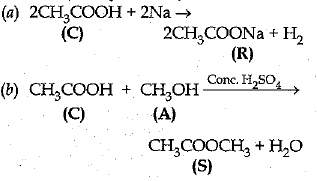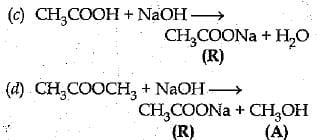Q. 27. (a) Name and state the rule to determine the direction of force experienced by a current carrying straight conductor placed in a uniform magnetic field which is perpendicular to it.
(b) Draw a labelled diagram of an electric motor.    (5 Mark)
Ans: (a) Direction of force acting on a current- carrying straight conductor due to a uniform perpendicular/magnetic field can be easily found by applying Fleming’s left-hand rule.
Fleming’s left-hand rule states that stretch the forefinger, the central finger and the thumb of your left-hand in mutually perpendicular directions. If the forefinger shows the direction of the magnetic field and the central finger that of the current, then the thumb wall point in the direction of force F.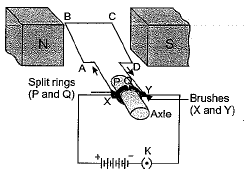(b) A labelled diagram of an electric motor is drawn here.

Q. 28. (i) Bacteria have a simpler body plan as compared to human beings who have a complex body organisation. Does it mean that human beings are more evolved than bacteria?
(ii) How can you conclude that birds are closely related to reptiles?
Or
(i) Study the following cross showing self-pollination in pea plant.
Fill in the blanks and answer the questions that follows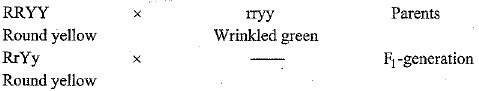(ii) Derive the combinations of characters expected in the F2 progeny? Also write the ratios,
(iii) According to you, what is responsible for the inheritance of traits?    (5 Mark)

Ans: (i) The terms simple and complex are used to classify organisms according to their evolutionary history. Simple organisms refer to those organisms that have simple structural and functional organisation and are considered primitive, whereas complex organisms refer to those organisms that have higher and complex levels of structural and functional organisation. These are more advanced and said to have arisen from simple organisms. Both bacteria and human beings perform all the activities of life in order to persist in their respective environment. Human beings have a more complex organisation and . differentiation. These features are absent in bacteria. Since, complexity and differentiation develop only through evolution, humans are said to be more evolved than bacteria.
(ii) Feathers provided insulation to dinosaurs in cold weather. Later in the evolutionary process, feathers were used for flight in birds. As dinosaurs were reptiles, it means that birds are closely related to reptiles.
Or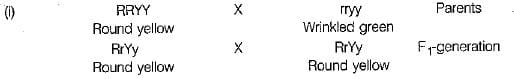(ii)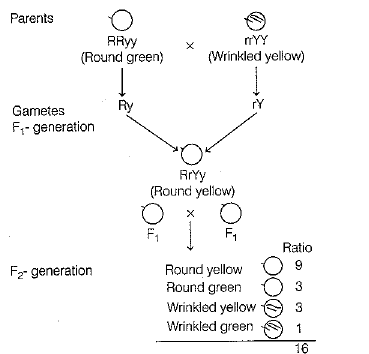Thus, the combination of characters obtained in the F2-generation and their respective ratios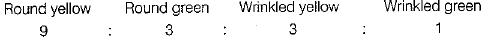(iii) Gene is the carrier that leads to the inheritance of traits. It is the part of a chromosome that controls the appearance of a set of hereditary characteristics.

Q. 29. An object is placed at a distance of 60 cm from a concave lens of focal length 30 cm.
(i) Use lens formula to find the distance of the image from the lens.
(ii) List four characteristics of the image (nature, position, size, erect/inverted) formed by the lens in this case.
(iii) Draw ray diagram to justify your answer of part (ii).
OR
(i) 4.5 cm needle is placed 12 cm away from a convex mirror of focal length 15 cm. Give the location of the image and the magnification.

Describe what happens as the needle is moved further from the mirror.
(ii) What kind of mirror is used in a solar furnace ? Give reason for using this mirror
(iii) One half of a convex lens is covered with a black paper. Will this lens produce a complete image of the object ? Justify your answer.    (5 Mark)

Ans: (i) Given u = - 60 cm, f =
Using lens formula,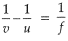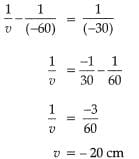Distance of the image will be 20 cm in front of lens.
(ii) Nature: Virtual
Position: Between F1 and O or on same side of lens where object is placed.
Size : Diminished and 1/3rd of object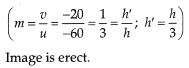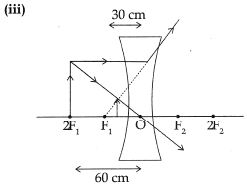Or
(i) u = -12 cm,
f = + 15 cm
Using mirror formula,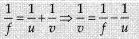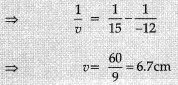Magnification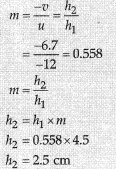As the needle moved farther from the mirror image moves to the focus and size of the image goes on decreasing
(ii) Concave mirrors are used in solar furnaces, as they concentrate solar energy in the focal plane and help in attaining high temperatures.
(iii) When one half of a convex lens is covered with a black paper, the lens will produce the complete image of the object, but the intensity of the image is reduced because rays only from the top portion of the lens are refracted and form the image.

Q. 30. An object is placed at the following distances from a concave mirror of focal length 10 cm:
(a) 8 cm
(b) 15 cm
(c) 20 cm
(d) 25 cm.
Which position of the object will produce ?
(i) a diminished real image?
(ii) a magnified real image?
(iii) a magnified virtual image.
(iv) an image of the same size as the object?
Or
(a) If a person wears lens of power - 6 D for distant vision and for correcting his near vision he needs a lens of +2 D. Determine the focal length of the lenses in both the case.
(b) Give reason for the following natural phenomenon:
(i) Stars twinkle
(ii) Planets do not twinkle
(iii) Stars appear raised in the sky    (5 Mark)
Ans:
Given focal length of mirror = 10 cm Therefore, distance of centre of curvature - 20 cm
(i) A diminished real image is formed by a concave mirror, when the object lies beyond C. Here C is at 20 cm. Therefore, the diminished real image will be formed when the object is at a distance greater than 20 cm, which in this problem is 25 cm. Hence, the position of the object for a diminished real image is 25 cm.
(ii) A magnified real image is formed by a concave mirror when the object is between F and C. Here F is at 10 cm and C is at 20 cm. Hence, the magnified real image will be formed when the object lies between 10 cm and 20 cm which in this problem is 15 cm. Therefore, the position of object for a magnified real image is 15 cm.
(iii) A concave mirror forms a magnified virtual image, when the object is within focus (F) at a distance less than focal length or less than 10 cm, which in this problem is 8 cm. Therefore, the position of object for a magnified virtual image is 8 cm
(iv) An image of the same size as object is formed by a concave mirror when the object lies at the centre of curvature (c). Here C is at 20 cm, therefore, the image of the same size as the object will be formed when the object is at 20 cm from the concave mirror. Thus, the position of the object for an image of same size as the-object is 20 cm.
Or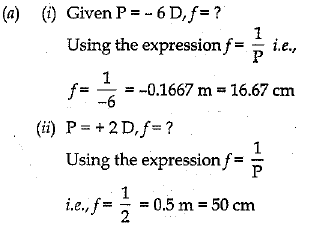(b) (i) The layers of the earth's atmosphere have different densities and hence different refractive index. This refractive index keeps on changing due to conventional currents in the atmosphere. When light from the star which can be approximated as a point source of light, enters such an atmosphere it seems to change its' position. The light coming from the stars, therefore, presents a quivering (shaking) appearance, thus, giving the impression as if the stars are twinkling.
(ii) The stars, although very large as compared to the planets, appear as tiny specks in the sky due to their immense distance from the earth as compared to the huge size of the planets. Thus, the stars appear as point sources of light and the planets appear as a large combination of such sources. Due to its seemingly larger size, the change in the direction of light coming from the planets is negligible as compared to that coming from the stars. Therefore, the planets do not seem to twinkle like stars.

The document Class 10 Science: CBSE Sample Question Paper (2019-20) - 10 Notes | Study CBSE Sample Papers For Class 10 - Class 10 is a part of the Class 10 Course CBSE Sample Papers For Class 10.
All you need of Class 10 at this link: Class 10

## CBSE Sample Papers For Class 10

174 docs|7 tests
 Use Code STAYHOME200 and get INR 200 additional OFF

## CBSE Sample Papers For Class 10

174 docs|7 tests

Track your progress, build streaks, highlight & save important lessons and more!

,

,

,

,

,

,

,

,

,

,

,

,

,

,

,

,

,

,

,

,

,

;